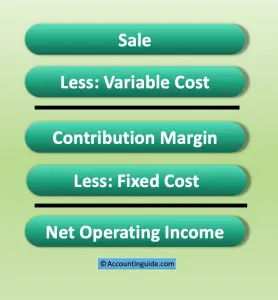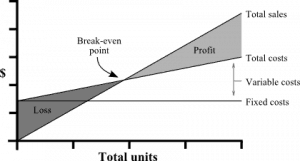# Break-Even Point

Break-Even Point in the point in which company generates total revenue equal to total cost. In other words, the company does not make any profit or loss. It is a useful ratio for management to set the target for more profit, any sale above the break-even will generate profit for the company. Break-even point can represent both sale amount (\$) or sale quantity (units).

It is very important because it helps the company to know their minimum sales which they have to make to recover their fixed cost. Any sale quantity above this will make a profit for the company.

It also helps us to understand the overall business performance whether the break-even point is too high or not. The higher the break-even point, the higher the risk we are facing.

## Formulas of Break-Even pointIn order to understand more clearly about these formulas, we have to understand the relationship between fixed cost and variable cost in the brief income statement as below:

There are a few ways to calculate the Break-Even point, such as:

Break-Even Point (units) = Fixed Cost / (Sale price per unit-VC per unit)

Break Even Point (units) = Fixed Cost/Contribution per unit

Break Even point (dollar) = Break-Even point (units) x selling price

Break-Even point (dollar) =   Fixed Cost /Contribution %

All of the formulas above will provide the same result as they find the specific point where contribution margin equal to fixed cost, and the profit/net income is zero.## Example

Company ABC manufactures premium pens and supplies them to the retail shops in the US.

We have set the selling price of \$ 5 per unit.

The variable cost per unit includes:

• Rubber                            \$ 1
• Worker wage                  \$ 1
• Other material                \$ 1

Monthly operating cost includes:

• Depreciation expense on building and machinery      \$ 5,000
• Rental fee on warehouse                                                \$ 2,000
• Salary for all staffs                                                         \$ 10,000
• Marketing expense                                                          \$ 3,000
• Other expenses                                                                \$ 1,000

Total                                                                                          \$ 21,000

Calculate the Break-Even Point

## Solution:

All materials and direct labor are the variable cost with a total of \$ 3 per unit.

All operating expenses are the fixed cost total of \$ 21,000, as we assume that they will not change due to production output.

Break-Even point = \$ 21,000 / (\$ 5 – \$ 3) = 10,500 units

The company needs to sell 10,500 units of pens to meet the break-even point. Any sale of more than 10,500 units will make a profit for the company.

If the sale is 10,500 units, the sale will be \$ 52,500 ( 10,500 units * \$ 5 per unit). The total cost will be \$ 52,500 [(10,500 units * \$ 3 per unit) + 21,000]. So the sale amount is equal to total cost, the company makes zero profit.